Welcome to Wall Street Prep! Use code at checkout for 15% off.# Capital Gains Yield (CGY)

Guide to Understanding the Capital Gains Yield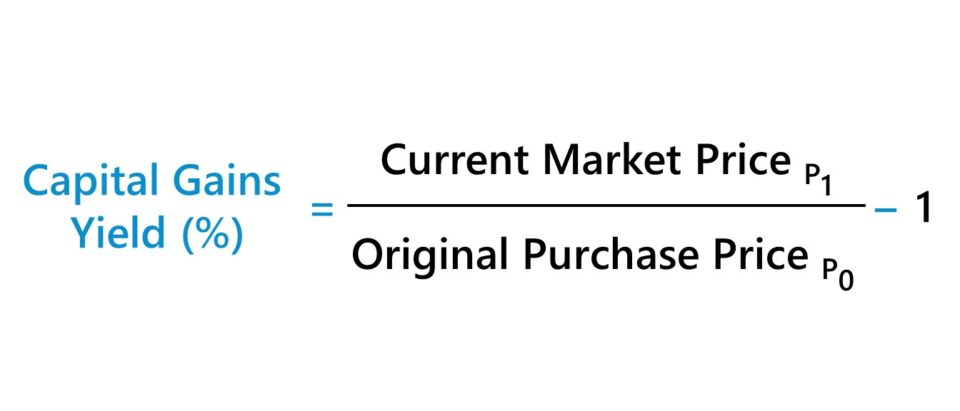## How to Calculate the Capital Gains Yield (Step-by-Step)

The capital gains yield, or “CGY”, calculates the change in the price of securities, expressed in the form of a percentage.

The returns of holding a publicly traded security, such as common shares, come from two sources.

1. Stock Price Appreciation
2. Shareholder Dividend Issuances

The capital gains yield calculation only takes into account the increase in the stock price and neglects any other income earned, such as via dividends.

• Capital Gain → If the share price has increased relative to the original price paid on the date of purchase, the stock price is said to have “appreciated” in value.
• Capital Loss → In contrast, if the share price has decreased in comparison to the purchase price, the stock price has “depreciated” in value and the yield would be negative.

The capital gains yield can be calculated using the following process:

• Step 1 → Determine the Original Purchase Price per Share
• Step 2 → Divide the Current Market Price by the Original Price Paid per Share
• Step 3 → Subtract 1 from the Resulting Figure

## Capital Gains Yield Formula

The capital gains yield formula is as follows.

Capital Gains Yield (%) = (Current Market Price ÷ Original Purchase Price)  1

## Capital Gains Yield vs. Dividend Yield

The other source of returns on public equities is income earned on the investment, such as the receipt of dividends on common stock.

Since the capital gains yield neglects any income received on an investment aside from share price appreciation, the metric can be used in conjunction with the dividend yield.

The dividend yield is the ratio between the dividend per share (DPS) and the current market share price.

Dividend Yield (%) = Dividend Per Share (DPS) ÷ Current Market Share Price

While certain companies will either not pay any shareholder dividends or opt to repurchase shares, mature companies with limited opportunities for growth frequently have long-term dividend programs to compensate their shareholder base.

Because corporate dividends are rarely cut once implemented, these so-called “dividend stocks” attract investors that prefer a steady stream of dividends over share price appreciation.

Given the reliance on the dividend payout returns, the share price of the company contributes less to the total return (and investors expect minimal movement in the stock price given the relatively stable fundamentals of the issuer).

## Short-Term and Long-Term Capital Gains Tax Rates (2022)

If the investment has been sold – assuming there was a profit (i.e. sale price > purchase price) – the “realized” capital gain becomes a form of taxable income.

On the other hand, an investment that has not yet been sold is an “unrealized” capital gain, which is not taxable.

The specific tax rate applied is jurisdiction-dependent among other factors, such as the individual’s taxable income and filing status.

The holding period can also impact the tax rate, where the applicable tax rate is reduced for assets held longer than one year compared to one sold before one year.

• Short-Term Capital Gain → Holding Period < 12 Month
• Long-Term Capital Gain → Holding Period > 12 Month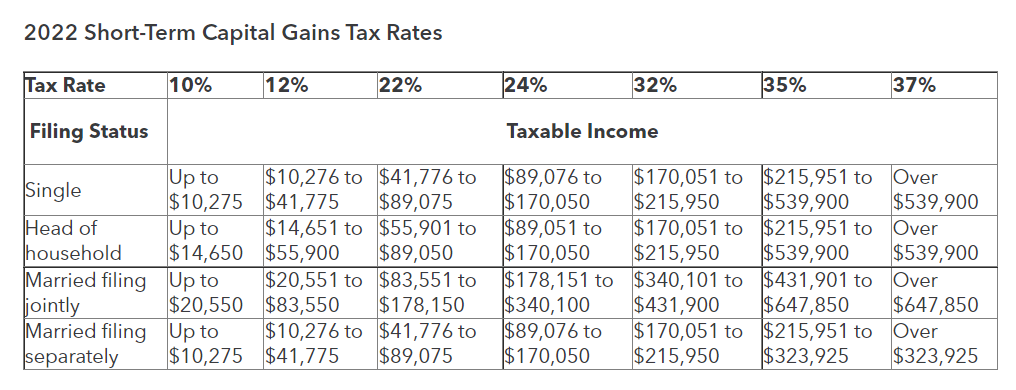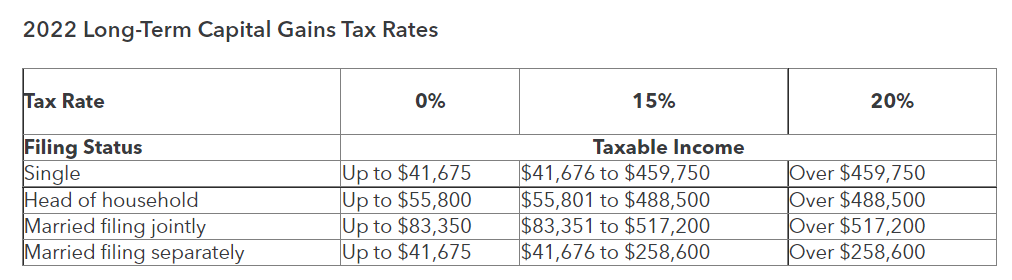Guide to the Capital Gains Tax Rate: Short-term vs. Long-term Capital Gains Taxes (Source: Intuit)

## Taxes and Dollar Cost Averaging Investing Strategy (DCA)

The cost basis of the shares purchased can change if the investor has purchased additional shares after the initial purchase.

For instance, one common strategy utilized by investors – often after the stock price declines below the original purchase price – is dollar cost averaging (DCA).

If the investor views the decline in price as an opportunity to increase the potential upside from the investment, i.e. a lower entry point, the DCA strategy can reduce the cost basis of the investment.

While using the reduced cost basis is technically more accurate for investors attempting to determine their actual yield, the tax implications are one factor to consider since each purchase of additional shares is viewed as a separate transaction.

## Capital Gains Yield Calculator (CGY)

We’ll now move on to a modeling exercise, which you can access by filling out the form below.Submitting...

## Capital Gains Yield Calculation Example

Suppose an investor purchased shares in a company at a cost basis of \$50.00 per share.

The share price of the underlying company rises to \$60.00 over the next year, which prompts the investor to exit the position at a net profit of \$10.00 per share.

• Original Purchase Price = \$50.00
• Current Market Value = \$60.00
• Capital Gain = \$60.00 – \$50.00 = \$10.00

The capital gains yield can be calculated by dividing the original purchase price per share by the current market value per share, minus 1.

• Capital Gains Yield (%) = (\$60.00 ÷ \$50.00) – 1 = 20%

In closing, the realized capital gains yield on the equity investment comes out to be a 20% return.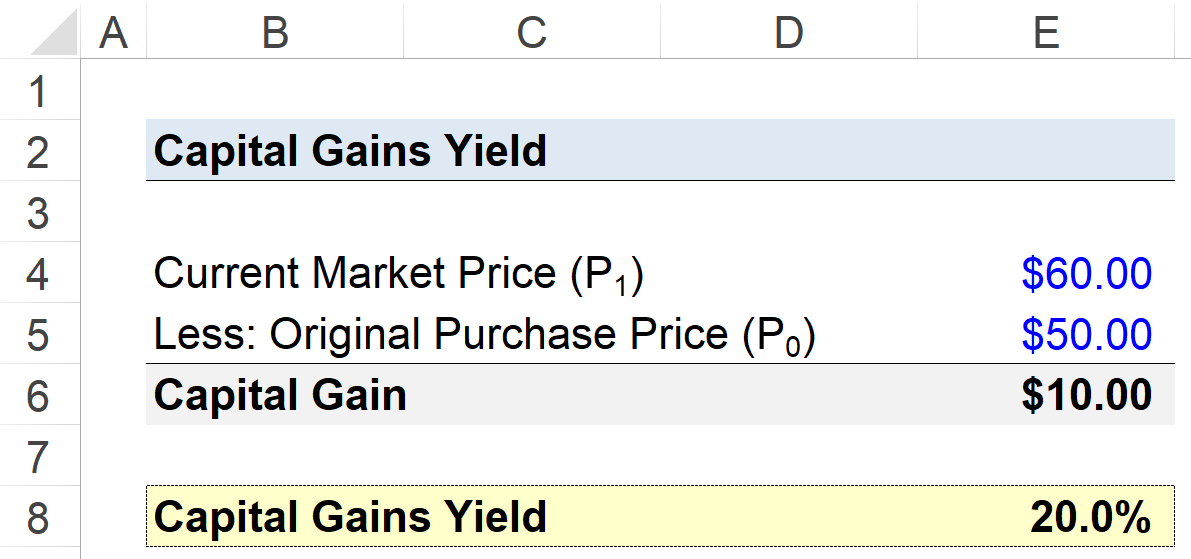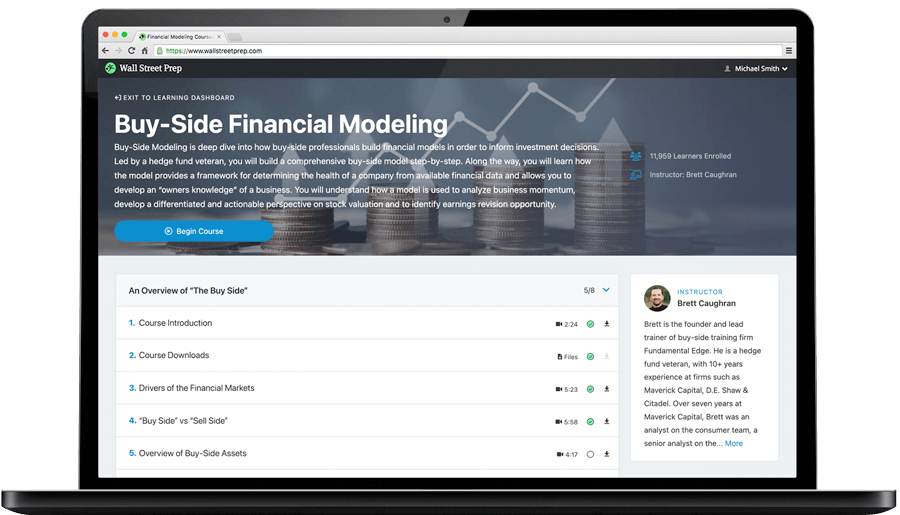Step-by-Step Online Course

### Learn Buy-Side (Hedge Fund) Modeling

Led by a former hedge fund PM (Maverick, Citadel, DE Shaw, Schonfeld), this program begins where financial modeling training ends — with a deep-dive into how buy-side analysts build financial models to make key investment decisions.